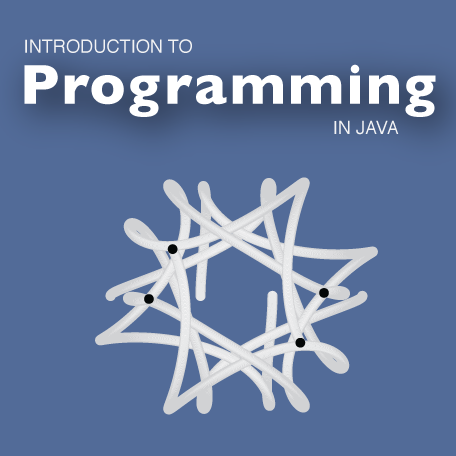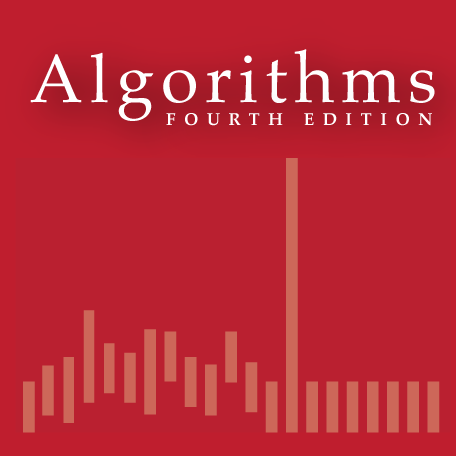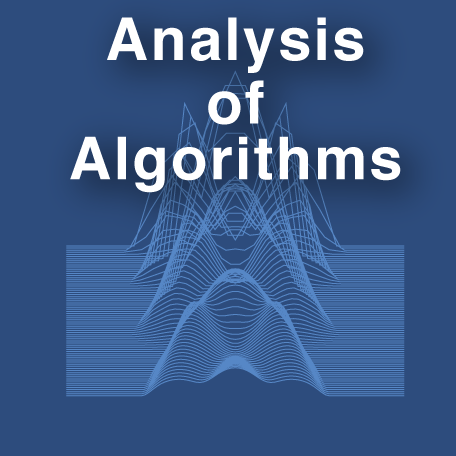# An Introduction to the Analysis of Algorithms

People who analyze algorithms have double happiness. First of all they experience the sheer beauty of elegant mathematical patterns that surround elegant computational procedures. Then they receive a practical payoff when their theories make it possible to get other jobs done more quickly and more economically.      D. E. Knuth

This booksite is (always) under construction.

## Textbook.

The textbook An Introduction to the Analysis of Algorithms (2nd edition) by Robert Sedgewick and Philippe Flajolet [ Amazon · Inform IT ] overviews the primary techniques used in the mathematical analysis of algorithms. The material covered draws from classical mathematical topics, including discrete mathematics, elementary real analysis, and combinatorics, as well as from classical computer science topics, including algorithms and data structures.
• Chapter 1: Analysis of Algorithms considers the general motivations for algorithmic analysis and relationships among various approaches to studying performance characteristics of algorithms.
• Chapter 2: Recurrence Relations concentrates on fundamental mathematical properties of various types of recurrence relations which arise frequently when analyzing an algorithm through a direct mapping from a recursive representation of a program to a recursive representation of a function describing its properties.
• Chapter 3: Generating Functions introduces a central concept in the average-case analysis of algorithms: generating functions — a necessary and natural link between the algorithms that are our objects of study and analytic methods that are necessary to discover their properties.
• Chapter 4: Asymptotic Approximations examines methods of deriving approximate solutions to problems or of approximating exact solutions, which allow us to develop concise and precise estimates of quantities of interest when analyzing algorithms.
• Chapter 5: Analytic Combinatorics introduces a modern approach to the study of combinatorial structures, where generating functions are the central object of study. This approach is the basis for the study of specific structures through the rest of the book.
• Chapter 6: Trees investigates properties of many different types of trees, fundamental structures that arise implicitly and explicitly in many practical algorithms. Our goal is to provide access to results from an extensive literature on the combinatorial analysis of trees, while at the same time providing the groundwork for a host of algorithmic applications.
• Chapter 7: Permutations surveys combinatorial properties of permutations (orderings of the numbers 1 through N) and shows how they relate in a natural way to fundamental and widely-used sorting algorithms.
• Chapter 8: String and Tries studies basic combinatorial properties of strings, sequences of characters or letters drawn from a fixed alphabet, and introduces algorithms that process strings ranging from fundamental methods at the heart of the theory of computation to practical text-processing methods with a host of important applications.
• Chapter 9: Words and Maps covers global properties of words (N-letter strings from an M-letter alphabet), which are well-studied in classical combinatorics (because they model sequences of independent Bernoulli trials) and in classical applied algorithmics (because they model input sequences for hashing algorithms). The chapter also covers random maps (N-letter words from an N-letter alphabet) and discusses relationships with trees and permutations.

## Booksite.

Reading a book and surfing the web are two different activities: This booksite is intended for your use while online (for example, while programming and while browsing the web); the textbook is for your use when initially learning new material and when reinforcing your understanding of that material (for example, when reviewing for an exam). The booksite consists of the following elements:

• Excerpts. A condensed version of the text narrative, for reference while online.

• Exercise solutions. Solutions to selected exercises.

• Java, Sage, and Python code. Validation of analytic results.

The book was first published in 1995. The second edition (2015) and this booksite aim to supplement the material in the text while still respecting the integrity of the original.

## Other resources.

To fully engage with this material, you will eventually want to download and use at least the following tools:

• StdJava code. The basic programming model that we developed for our books Introduction to Programming (in Java) and Algorithms, 4th Edition. Available at the Algs4 booksite.

• $\TeX$. Classical math typsetting software. Get started at the $\TeX$ user's group website.

• MathJax. Mechanism for embedding math in web pages. No need to download, just link to their site. See the MathJax home page. MathJax test: When $a \ne 0$, there are two solutions to $ax^2 + bx + c = 0$ and they are $$x = {-b \pm \sqrt{b^2-4ac} \over 2a}.$$ If you are using Mac OS X Lion and Safari/Chrome in early 2012 and this math looks bad, try disabling the STIX fonts in your FontBook.